# Square and circles

Square with sides 83 cm is circumscribed and inscribed with circles. Determine the radiuses of both circles.

r =  41.5 cm
R =  58.69 cm

### Step-by-step explanation: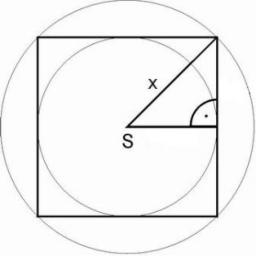Did you find an error or inaccuracy? Feel free to write us. Thank you!Tips to related online calculators
Pythagorean theorem is the base for the right triangle calculator.

#### You need to know the following knowledge to solve this word math problem:

We encourage you to watch this tutorial video on this math problem:

## Related math problems and questions:

• Circles 2Calculate the area bounded by the circumscribed and inscribed circle in triangle with sides 12 cm, 14 cm, 18 cm.
• CirclesThe area of a circle inscribed in a square is 14. What is the area of a circle circumscribed around a square?
• Rhombus and inscribed circleIt is given a rhombus with side a = 6 cm and the radius of the inscribed circle r = 2 cm. Calculate the length of its two diagonals.
• Circumscribing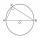Determine the radius of the circumscribed circle to the right triangle with legs 6 cm and 3 cm.
• Two circles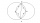Two circles with the same radius r = 1 are given. The center of the second circle lies on the circumference of the first. What is the area of a square inscribed in the intersection of given circles?
• Rectangle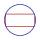The rectangle is 18 cm long and 10 cm wide. Determine the diameter of the circle circumscribed to the rectangle.
• Square circles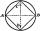Calculate the length of the described and inscribed circle to the square ABCD with a side of 5cm.
• RT and circlesSolve right triangle if the radius of inscribed circle is r=9 and radius of circumscribed circle is R=23.
• Inscribed circleXYZ is right triangle with right angle at the vertex X that has inscribed circle with a radius 5 cm. Determine area of the triangle XYZ if XZ = 14 cm.
• Annular areaThe square with side a = 1 is inscribed and circumscribed by circles. Find the annular area.
• RT - inscribed circleIn a rectangular triangle has sides lengths> a = 30cm, b = 12.5cm. The right angle is at the vertex C. Calculate the radius of the inscribed circle.
• Circumscribed circle to squareFind the length of a circle circumscribing a square of side 10 cm. Compare it to the perimeter of this square.
• Concentric circlesIn the circle with diameter, 19 cm is constructed chord 9 cm long. Calculate the radius of a concentric circle that touches this chord.
• Reverse Pythagorean theoremGiven are lengths of the sides of the triangles. Decide which one is rectangular: Δ ABC: 77 dm, 85 dm, 36 dm ... Δ DEF: 55 dm, 82 dm, 61 dm ... Δ GHI: 24 mm, 25 mm, 7 mm ... Δ JKL: 32 dm, 51 dm, 82 dm ... Δ MNO: 51 dm, 45 dm,
• Concentric circles and chordIn a circle with a diameter d = 10 cm, a chord with a length of 6 cm is constructed. What radius have the concentric circle while touch this chord?
• Desribed circle to rectangle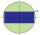Rectangle with sides 6 cm and 4 cm was circumscribed circle. What part of the content of the circle determined by the circumscribed circle occupies a rectangle? Express in perctentages(%).
• RhombusIt is given a rhombus of side length a = 19 cm. Touchpoints of inscribed circle divided his sides into sections a1 = 5 cm and a2 = 14 cm. Calculate the radius r of the circle and the length of the diagonals of the rhombus.